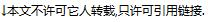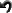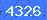# 锁相环环路滤波器计算器## 三阶电荷泵锁相环环路滤波器计算器function rechnen() { var f_ref, f_out, loopbw, Kvco, Icp, att, phi; pi = 3.141592653 ; f_ref = document.generator.f_ref.value * 1; f_out = document.generator.f_out.value * 1; loopbw = document.generator.loopbw.value * 1; Kvco = document.generator.Kvco.value * 1; Icp = document.generator.Icp.value * 1; att = document.generator.att.value * 1; phi = document.generator.phi.value * 1; n = Math.round( 1000 * f_out / f_ref) / 1000 ; t1 = ((1/Math.cos(pi*phi/180) - Math.tan(phi*pi/180))/( 2*pi*loopbw )); t3 = Math.sqrt((Math.pow(10,(att/20))-1)/(4*pi*pi*f_ref*f_ref)); omega =((Math.tan(pi*phi/180)*(t1+t3))/((t1+t3)*(t1+t3)+t1*t3))*(Math.sqrt(1+((t1+t3)*(t1+t3)+t1*t3)/(Math.tan(pi*phi/180)*(t1+t3)*Math.tan(pi*phi/180)*(t1+t3)))-1); t2 =1/(omega*omega*(t1+t3)) c1 =(t1/t2)*((Icp*0.001*Kvco*1000000)/(omega*omega*n))* Math.sqrt((1+omega*omega*t2*t2)/((1+omega*omega*t1*t1)*(1+omega*omega*t3*t3)))*1000000000000; c2 =c1*((t2/t1)-1); r2 = t2*1000000000000/c2 ; c3 = c1/10 ; r3 = t3*1000000000000/c3 ; c1 = Math.ceil(c1)/1000; c2 = Math.ceil(c2)/1000; c3 = Math.ceil(c3)/1000; r2 = Math.ceil(r2); r3 = Math.ceil(r3); document.generator.n.value = n; document.generator.c1.value = c1; document.generator.c2.value = c2; document.generator.c3.value = c3; document.generator.r2.value = r2; document.generator.r3.value = r3; }

 输出频率 [Hz] 参考频率 [Hz] 环路带宽 [Hz] 相裕度 [deg] 压控振荡器的增益 [MHz/V] 电荷泵的增益 [mA/2*Π*rad] 参考衰减 [dB]

 预分频器 C1 [nF] C2 [nF] C3 [nF] R2 [Ω] R3 [Ω]返回首页

 发表评论

 验证码:看不清楚,点击刷新

• 很好　匿名 于2021-10-19 14:58:10发布
• 好资料　bh1aq 于2021-10-15 09:42:33发布
• gsddhfshf　搞不懂 于2021-10-08 19:35:51发布
• 的确很实用　匿名 于2021-09-24 13:35:47发布
• 非常不错！谢谢！　匿名 于2021-09-19 22:55:08发布
• 20点是多少费用　山青竹 于2021-09-16 17:19:25发布
• 很好　海之韵 于2021-09-06 01:22:55发布
• 感谢分享，很有价值的资料　18032887003 于2021-08-30 10:47:42发布